﻿ INVESTIGATION OF REFLECTION BANDS OF 1D ANNULAR PHOTONIC CRYSTAL CONTAINING DOUBLE NEGATIVE INDEX MATERIAL AND NON-MAGNETIZED PLASMA

# INVESTIGATION OF REFLECTION BANDS OF 1D ANNULAR PHOTONIC CRYSTAL CONTAINING DOUBLE NEGATIVE INDEX MATERIAL AND NON-MAGNETIZED PLASMA

A. Aghajamali a, S.K. Srivastava b, and C. Nayak cd

a Department of Physics and Astronomy, Curtin University, Perth, WA 6102, Australia

b Department of Physics, Amity Institute of Applied Sciences, Amity University Uttar Pradesh, Noida, India

c Department of Electronics and Communication Engineering, SRM Institute of Science and Technology, Chennai-603203, India

d Department of Communication Engineering, School of Electronics Engineering, Vellore Institute of Technology, Vellore, Tamil Nadu 632014, India
Email: sanjeev17th@yahoo.co.in

Received 8 March 2022; revised 1 July 2022; accepted 8 August 2022

In this paper, optical reflectance properties of an annular photonic crystal (APC) composed of alternate layers of double negative (DNG) material and a non-magnetized plasma (NMP) layer, immersed in free space, have been theoretically investigated and studied. The reflectance spectra of the annular PC have been obtained by employing the transfer matrix method (TMM) for the cylindrical waves in the case of TE-polarized wave only. It has been found that the spectral position and width of the reflection bands of APC are greatly influenced by the variation in the thickness of DNG metamaterial and NMP layer, respectively. Interestingly, it is observed that the presence of NMP layer causes the increase in photonic band gap (PBG) whereas the DNG layer reduces the PBG. Further, the effect of azimuthal mode number (m) on the reflectance spectra shows that for m > 0, splitting in the reflection bands occurs at the frequency corresponding to the zero permeability value of DNG metamaterial layer. Moreover, with the increase in azimuthal mode number one PBG is red-shifted and the second one is blue-shifted. Finally, the effect of change in the starting radius parameter of curved surface and plasma electron density on the reflectance spectra of APC has also been studied and very interesting results have been observed.

Keywords: annular photonic crystal, circular photonic crystal, cylindrical Bragg waves, reflectance spectra, double negative index material, non-magnetized plasma, transfer matrix method

## 1. Introduction

The periodic profile of alternate dielectric constant of the material on the optical wavelength scale has the  properties of controlling and manipulating the flow of light wave and is called photonic crystal (PC) . When the optical wave propagates through such novel class of optical materials, then a  certain range of frequencies (or wavelengths) are prohibited. The forbidden frequency (or wavelength) range is called stop band or photonic band gap (PBG) and arises due to interference of Bragg scattering. Since in the  PC propagation of light wave can be controlled and manipulated very effectively, therefore, it has various applications in the field of photonics and optoelectronics .

The introduction of double negative (DNG) materials in designing and fabrication of photonic crystals has focused much interest in the scientific community during the  last two decades . DNG materials, also called negative index materials (NIMs) or left-handed materials (LHMs), are artificial composites with a  simultaneous negative permittivity and permeability. DNG material was theoretically investigated by Veselago  and experimentally demonstrated by Smith et al. . When the electromagnetic wave propagates through the DNG material, the direction of Poynting vector S  =  E  ×  H becomes opposite to that of the wave vector k, so that k, E and H form a left-handed set of vectors. Thus, when such types of metamaterial are used in a PC structure, a very distinct feature is observed .

PCs containing the non-magnetized and magnetized plasma have also been the subject of attraction to researchers and scientists in recent years. By changing the  parameters of plasma materials, the position and width of transmission and reflection bands can be tuned at a particular frequency and a different range of frequencies .

In recent times, the propagation of electromagnetic wave in a  cylindrical multilayer structure (CMS) has also received much attention and generated lots of interest among the scientific community . A photonic crystal having a periodic cylindrical multilayer structure is called a circular photonic crystal (CPC) or an annular photonic crystal (APC). Due to the technological advancement of modern fabrication technique it is possible to design and fabricate a photonic crystal in different geometrical shapes  . An annular Bragg laser (ABR) or resonators consisting of ABRs surrounding a radial defect have been developed, which shows a specific feature of vertical emission. Such types of lasers have a better sensing ability in the  biological and chemical function than planar PC resonators, in addition to the  application in the optical communication system .

Kaliteevski et al.  have developed the  version of transfer matrix method (TMM), like in a planar PC that is applied to analyze the propagation of electromagnetic wave in APC. The optical characteristics of APC (or CPC) composed of different types of materials have been studied by many researchers .

In this work, we theoretically investigate and study the reflectance properties of an annular photonic crystal (APC) composed of alternate layers of DNG material and non-magnetized plasma immersed in free space. In order to obtain reflectance spectra we use the  transfer matrix method (TMM) for cylindrical waves that was developed by Kaliteevski et al. . We will study the effects of change in the  thickness of DNG metamaterial and NMP layer, the azimuthal mode number (m), the  electron density of plasma material and the starting radius (ρ0) on the reflectance spectra of the proposed APC structure for the TE-wave only.

## 2. Theoretical modelling and formulation

The schematic diagram of one-dimensional annular photonic crystal is illustrated in Fig. 1. The  structure consists of the  inner core region with the refractive index n0 (starting medium) and the  starting radius ρ0. Green and orange colours represent the DNG metamaterial and NMP layer, respectively. The first layer A has the refractive index n1 (permittivity ε1 and permeability µ1) with the thickness dA and radius ρ1 whereas the second layer B has the refractive index n2, thickness dB and radius ρ2, respectively. The outer region (final medium) of the structure has the refractive index nf with the radius ρf.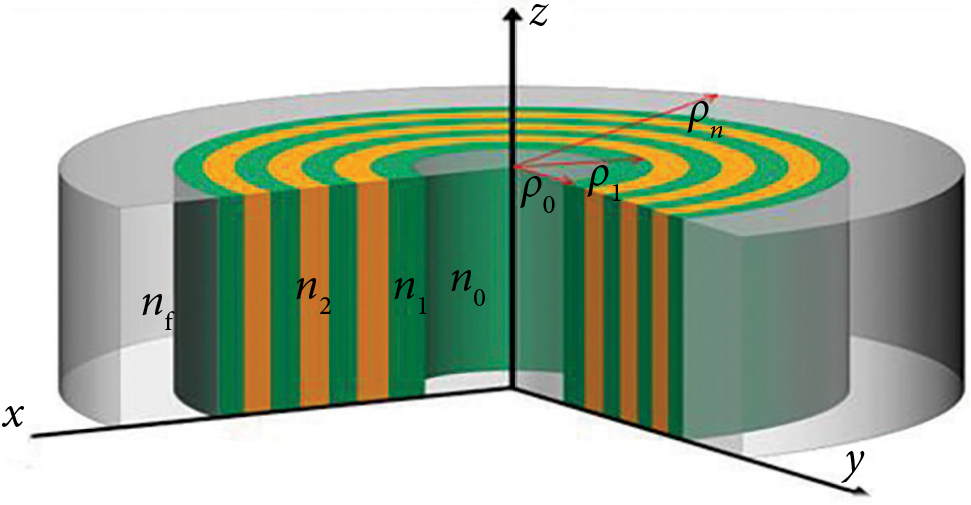Fig. 1. Schematic diagram of the  APC structure consisting of the  inner core region with the  refractive index n0 and starting radius ρ0. Green and orange colours represent the  DNG metamaterial and the NMP layer, respectively, having refractive indices, thicknesses and radius n1, n2, dA, dB and radii ρ1 and ρ2, respectively. The  outer region (final medium) of the structure has refractive index nf with radius ρf.

Here we consider the physically realizable DNG metamaterial from Ref. , with effective permittivity ε1 and permeability µ1 given by

${\epsilon }_{1}\left(f\right)=1+\frac{{5}^{2}}{{\left(0.9\right)}^{2}-{f}^{2}-\text{i}f{\gamma }_{\text{e}}}+\frac{{10}^{2}}{{\left(10.5\right)}^{2}-{f}^{2}-\text{i}f{\gamma }_{\text{e}}}\text{\hspace{0.17em}}\text{\hspace{0.17em}}\text{\hspace{0.17em}}\text{\hspace{0.17em}}\text{\hspace{0.17em}}\text{\hspace{0.17em}}\text{\hspace{0.17em}}\text{\hspace{0.17em}}\text{\hspace{0.17em}}\text{\hspace{0.17em}}\text{\hspace{0.17em}}\text{\hspace{0.17em}}\text{\hspace{0.17em}}\left(1\right)$
${\mu }_{1}\left(f\right)=1+\frac{{3}^{2}}{{\left(0.902\right)}^{2}-{f}^{2}-\text{i}f{\gamma }_{\text{m}}},\text{\hspace{0.17em}}\text{\hspace{0.17em}}\text{\hspace{0.17em}}\text{\hspace{0.17em}}\text{\hspace{0.17em}}\text{\hspace{0.17em}}\text{\hspace{0.17em}}\text{\hspace{0.17em}}\text{\hspace{0.17em}}\left(2\right)$

where f is the angular frequency, γe and γm are electric and magnetic damping frequencies (also called electric and magnetic loss factors) measured in GHz.

The refractive index of non-magnetized plasma is given by the equation 

${n}_{2}\left(f\right)=\sqrt{{\epsilon }_{2}\text{\hspace{0.17em}}\left(f\right)}=\sqrt{1-\frac{{\left({f}_{pe}\right)}^{2}}{{f}^{2}},}\text{\hspace{0.17em}}\text{\hspace{0.17em}}\text{\hspace{0.17em}}\text{\hspace{0.17em}}\text{\hspace{0.17em}}\text{\hspace{0.17em}}\text{\hspace{0.17em}}\text{\hspace{0.17em}}\text{\hspace{0.17em}}\text{\hspace{0.17em}}\text{\hspace{0.17em}}\text{\hspace{0.17em}}\text{\hspace{0.17em}}\text{\hspace{0.17em}}\left(3\right)$

where ${f}_{pe}\text{\hspace{0.17em}}=\text{\hspace{0.17em}}{\left(\frac{{n}_{e}{e}^{2}}{4{\pi }^{2}{m}_{e}{\epsilon }_{0}}\right)}^{1}{2}}$ is the plasma frequency, ne is the electron plasma density, and me and e are the electron mass and charge, respectively.

It is assumed that the  cylindrical wave would diverge from the axis of symmetry ρ = 0 and then interfere normally on the  first interface at ρ  =  ρ0. The reflectance at the first circular boundary ρ = ρ0 is obtained by employing the transfer matrix method (TMM) in the cylindrical Bragg wave [42, 45] which is the most useful technique to analyze the transmission and reflection properties of PC structure.

Assuming that the  time dependent part of all the electromagnetic fields is exp(jωt), the source-free two curl Maxwell’s equations are given as

$\nabla ×E=-\text{i}\omega \mu H,\text{\hspace{0.17em}}\text{\hspace{0.17em}}\text{\hspace{0.17em}}\text{\hspace{0.17em}}\text{\hspace{0.17em}}\text{\hspace{0.17em}}\text{\hspace{0.17em}}\text{\hspace{0.17em}}\text{\hspace{0.17em}}\text{\hspace{0.17em}}\text{\hspace{0.17em}}\text{\hspace{0.17em}}\text{\hspace{0.17em}}\text{\hspace{0.17em}}\text{\hspace{0.17em}}\text{\hspace{0.17em}}\text{\hspace{0.17em}}\text{\hspace{0.17em}}\text{\hspace{0.17em}}\text{\hspace{0.17em}}\text{\hspace{0.17em}}\text{\hspace{0.17em}}\text{\hspace{0.17em}}\left(4\right)$

By using the method of separation of variables, we can get the solution for Ez which is expressed as

$\nabla ×H=\text{i}\omega \epsilon E.\text{\hspace{0.17em}}\text{\hspace{0.17em}}\text{\hspace{0.17em}}\text{\hspace{0.17em}}\text{\hspace{0.17em}}\text{\hspace{0.17em}}\text{\hspace{0.17em}}\text{\hspace{0.17em}}\text{\hspace{0.17em}}\text{\hspace{0.17em}}\text{\hspace{0.17em}}\text{\hspace{0.17em}}\text{\hspace{0.17em}}\text{\hspace{0.17em}}\text{\hspace{0.17em}}\text{\hspace{0.17em}}\text{\hspace{0.17em}}\text{\hspace{0.17em}}\text{\hspace{0.17em}}\text{\hspace{0.17em}}\text{\hspace{0.17em}}\text{\hspace{0.17em}}\text{\hspace{0.17em}}\text{\hspace{0.17em}}\text{\hspace{0.17em}}\text{\hspace{0.17em}}\text{\hspace{0.17em}}\text{\hspace{0.17em}}\text{\hspace{0.17em}}\text{\hspace{0.17em}}\left(5\right)$

There are two possible modes (or polarizations) including TE and TM modes for the  cylindrical Bragg wave. Ez, Hφ and Hρ are the non-zero fields for the  TE-wave and satisfy the  following three equations in each single layer

$\frac{1}{\rho }\text{\hspace{0.17em}}\frac{\partial {E}_{z}}{\partial \phi }\text{\hspace{0.17em}}=\text{\hspace{0.17em}}-\text{i}\omega \mu {H}_{\rho },\text{\hspace{0.17em}}\text{\hspace{0.17em}}\text{\hspace{0.17em}}\text{\hspace{0.17em}}\text{\hspace{0.17em}}\text{\hspace{0.17em}}\text{\hspace{0.17em}}\text{\hspace{0.17em}}\text{\hspace{0.17em}}\text{\hspace{0.17em}}\text{\hspace{0.17em}}\text{\hspace{0.17em}}\text{\hspace{0.17em}}\text{\hspace{0.17em}}\text{\hspace{0.17em}}\text{\hspace{0.17em}}\text{\hspace{0.17em}}\text{\hspace{0.17em}}\text{\hspace{0.17em}}\text{\hspace{0.17em}}\text{\hspace{0.17em}}\text{\hspace{0.17em}}\text{\hspace{0.17em}}\text{\hspace{0.17em}}\text{\hspace{0.17em}}\text{\hspace{0.17em}}\text{\hspace{0.17em}}\text{\hspace{0.17em}}\text{\hspace{0.17em}}\text{\hspace{0.17em}}\text{\hspace{0.17em}}\text{\hspace{0.17em}}\text{\hspace{0.17em}}\text{\hspace{0.17em}}\text{\hspace{0.17em}}\text{\hspace{0.17em}}\left(6a\right)$
$\frac{\partial {E}_{z}}{\partial \rho }\text{\hspace{0.17em}}=\text{\hspace{0.17em}}-\text{i}\omega \mu {H}_{\phi },\text{\hspace{0.17em}}\text{\hspace{0.17em}}\text{\hspace{0.17em}}\text{\hspace{0.17em}}\text{\hspace{0.17em}}\text{\hspace{0.17em}}\text{\hspace{0.17em}}\text{\hspace{0.17em}}\text{\hspace{0.17em}}\text{\hspace{0.17em}}\text{\hspace{0.17em}}\text{\hspace{0.17em}}\text{\hspace{0.17em}}\text{\hspace{0.17em}}\text{\hspace{0.17em}}\text{\hspace{0.17em}}\text{\hspace{0.17em}}\text{\hspace{0.17em}}\text{\hspace{0.17em}}\text{\hspace{0.17em}}\text{\hspace{0.17em}}\text{\hspace{0.17em}}\text{\hspace{0.17em}}\text{\hspace{0.17em}}\text{\hspace{0.17em}}\text{\hspace{0.17em}}\text{\hspace{0.17em}}\text{\hspace{0.17em}}\text{\hspace{0.17em}}\text{\hspace{0.17em}}\text{\hspace{0.17em}}\text{\hspace{0.17em}}\text{\hspace{0.17em}}\text{\hspace{0.17em}}\text{\hspace{0.17em}}\text{\hspace{0.17em}}\text{\hspace{0.17em}}\text{\hspace{0.17em}}\text{\hspace{0.17em}}\text{\hspace{0.17em}}\text{\hspace{0.17em}}\left(6b\right)$
$\frac{\partial \left(\rho {H}_{\phi }\right)}{\partial \rho }\text{\hspace{0.17em}}-\text{\hspace{0.17em}}\frac{\partial {H}_{\rho }}{\partial \phi }\text{\hspace{0.17em}}\text{i}\omega \epsilon \rho {E}_{z}.\text{\hspace{0.17em}}\text{\hspace{0.17em}}\text{\hspace{0.17em}}\text{\hspace{0.17em}}\text{\hspace{0.17em}}\text{\hspace{0.17em}}\text{\hspace{0.17em}}\text{\hspace{0.17em}}\text{\hspace{0.17em}}\text{\hspace{0.17em}}\text{\hspace{0.17em}}\text{\hspace{0.17em}}\text{\hspace{0.17em}}\text{\hspace{0.17em}}\text{\hspace{0.17em}}\text{\hspace{0.17em}}\text{\hspace{0.17em}}\text{\hspace{0.17em}}\text{\hspace{0.17em}}\text{\hspace{0.17em}}\text{\hspace{0.17em}}\text{\hspace{0.17em}}\text{\hspace{0.17em}}\text{\hspace{0.17em}}\text{\hspace{0.17em}}\text{\hspace{0.17em}}\left(6c\right)$

The governing equation for Ez can be obtained from Eqs. (6a–c) and is given by

$\begin{array}{c}\rho \frac{\partial }{\partial \rho }\left(\rho \frac{\partial {E}_{z}}{\partial \rho }\right)\text{\hspace{0.17em}}-\text{\hspace{0.17em}}{\rho }^{2}\text{\hspace{0.17em}}\frac{1}{\mu }\text{\hspace{0.17em}}\frac{\partial \mu }{\partial \rho }\text{\hspace{0.17em}}\frac{\partial {E}_{z}}{\partial \rho }\\ +\text{\hspace{0.17em}}\frac{\partial }{\partial \phi }\text{\hspace{0.17em}}\left(\frac{\partial {E}_{z}}{\partial \phi }\right)\text{\hspace{0.17em}}+\text{\hspace{0.17em}}{\omega }^{2}\text{\hspace{0.17em}}\mu \epsilon {\rho }^{2}{E}_{z}\text{\hspace{0.17em}}0\end{array}\text{\hspace{0.17em}}\text{\hspace{0.17em}}\text{\hspace{0.17em}}\text{\hspace{0.17em}}\text{\hspace{0.17em}}\text{\hspace{0.17em}}\text{\hspace{0.17em}}\text{\hspace{0.17em}}\text{\hspace{0.17em}}\text{\hspace{0.17em}}\text{\hspace{0.17em}}\text{\hspace{0.17em}}\text{\hspace{0.17em}}\text{\hspace{0.17em}}\text{\hspace{0.17em}}\text{\hspace{0.17em}}\left(7\right)$

By using the method of separation of variables, we can get the solution for Ez which is expressed as

$\begin{array}{c}{E}_{z}\left(\rho ,\text{\hspace{0.17em}}\phi \right)\text{\hspace{0.17em}}=\text{\hspace{0.17em}}V\left(\rho \right)\text{\hspace{0.17em}}\varphi \text{\hspace{0.17em}}\left(\phi \right)\\ =\text{\hspace{0.17em}}\left[A{J}_{m}\text{\hspace{0.17em}}\left(kp\right)\text{\hspace{0.17em}}+\text{\hspace{0.17em}}B{Y}_{m}\text{\hspace{0.17em}}\left(kp\right)\text{\hspace{0.17em}}exp\left(im\phi \right),\end{array}\text{\hspace{0.17em}}\text{\hspace{0.17em}}\text{\hspace{0.17em}}\text{\hspace{0.17em}}\text{\hspace{0.17em}}\text{\hspace{0.17em}}\text{\hspace{0.17em}}\text{\hspace{0.17em}}\text{\hspace{0.17em}}\text{\hspace{0.17em}}\text{\hspace{0.17em}}\text{\hspace{0.17em}}\text{\hspace{0.17em}}\text{\hspace{0.17em}}\text{\hspace{0.17em}}\text{\hspace{0.17em}}\text{\hspace{0.17em}}\text{\hspace{0.17em}}\left(8\right)$

where A and B are considered as constants. Jm and Ym are the Bessel function and Neumann function, respectively, m is the azimuthal number, $k=\omega \sqrt{\mu \epsilon }$ is the wave number of the medium and ω is the angular frequency.

The azimuthal part of the magnetic field Hφ can be obtained from Eq. (6b) and is given by

$\begin{array}{c}{H}_{\phi \text{\hspace{0.17em}}}\left(p,\text{\hspace{0.17em}}\phi \right)\text{\hspace{0.17em}}=\text{\hspace{0.17em}}U\left(\rho \right)\phi \left(\phi \right)\\ =\text{\hspace{0.17em}}-\text{ip}\text{\hspace{0.17em}}\left[A{J}_{m}\text{\hspace{0.17em}}\left(k\rho \right)\text{\hspace{0.17em}}+\text{\hspace{0.17em}}B{Y}_{m}\text{\hspace{0.17em}}\left(k\rho \right)\right]\text{\hspace{0.17em}}exp\left(im\phi \right).\end{array}\text{\hspace{0.17em}}\text{\hspace{0.17em}}\text{\hspace{0.17em}}\text{\hspace{0.17em}}\text{\hspace{0.17em}}\text{\hspace{0.17em}}\text{\hspace{0.17em}}\text{\hspace{0.17em}}\text{\hspace{0.17em}}\text{\hspace{0.17em}}\text{\hspace{0.17em}}\text{\hspace{0.17em}}\text{\hspace{0.17em}}\text{\hspace{0.17em}}\text{\hspace{0.17em}}\text{\hspace{0.17em}}\text{\hspace{0.17em}}\left(9\right)$

In Eq. (9), $p=\sqrt{\mu /\epsilon }$ represents the intrinsic admittance/impedance of the medium. The single layer matrix relationship corresponding to the electric field and magnetic field at its two interfaces can be obtained with the help of Eqs. (8) and (9).

The matrix for the first layer M1 with the refractive index n1 and interfaces at ρ = ρ0 and ρ1 can be expressed as 

$\left[\begin{array}{c}V\left({\rho }_{1}\right)\\ U\left({\rho }_{1}\right)\end{array}\right]\text{\hspace{0.17em}}=\text{\hspace{0.17em}}{M}_{1}\text{\hspace{0.17em}}\left[\begin{array}{c}V\left({\rho }_{0}\right)\\ U\left({\rho }_{0}\right)\end{array}\right],\text{\hspace{0.17em}}\text{\hspace{0.17em}}\text{\hspace{0.17em}}\text{\hspace{0.17em}}\text{\hspace{0.17em}}\text{\hspace{0.17em}}\text{\hspace{0.17em}}\text{\hspace{0.17em}}\text{\hspace{0.17em}}\text{\hspace{0.17em}}\text{\hspace{0.17em}}\text{\hspace{0.17em}}\text{\hspace{0.17em}}\text{\hspace{0.17em}}\text{\hspace{0.17em}}\text{\hspace{0.17em}}\text{\hspace{0.17em}}\text{\hspace{0.17em}}\text{\hspace{0.17em}}\text{\hspace{0.17em}}\text{\hspace{0.17em}}\text{\hspace{0.17em}}\text{\hspace{0.17em}}\text{\hspace{0.17em}}\text{\hspace{0.17em}}\text{\hspace{0.17em}}\left(10\right)$

where the single layer matrix M1 is given by

${M}_{1}=\left[\begin{array}{ll}{m}_{11}\hfill & {m}_{12}\hfill \\ {m}_{11}\hfill & {m}_{22}\hfill \end{array}\right].$

The matrix elements of M1 are given by

$\begin{array}{rr}\hfill & \hfill {m}_{11}=\frac{\pi }{2}{k}_{1}{\rho }_{0}\left[{Y}_{m}^{\text{'}}\left({k}_{1}{\rho }_{0}\right){J}_{m}\left({k}_{1}{\rho }_{1}\right)\\ \hfill & \hfill -{J}_{m}^{\text{'}}\left({k}_{1}{\rho }_{0}\right){Y}_{m}\left({k}_{1}{\rho }_{1}\right)\right]\\ \hfill & \hfill {m}_{12}=i\frac{\pi }{2}\frac{{k}_{1}}{{\rho }_{1}}{\rho }_{0}\left[{J}_{m}\left({k}_{1}{\rho }_{0}\right){Y}_{m}\left({k}_{1}{\rho }_{1}\right)\\ \hfill & \hfill -{Y}_{m}\left({k}_{1}{\rho }_{0}\right){J}_{m}\left({k}_{1}{\rho }_{1}\right)\right],\\ \hfill & \hfill {m}_{21}=i\frac{\pi }{2}{k}_{1}{\rho }_{0}{p}_{1}\left[{Y}_{m}^{\text{'}}\left({k}_{1}{\rho }_{0}\right){J}_{m}^{\text{'}}\left({k}_{1}{\rho }_{1}\right)\\ \hfill & \hfill -{J}_{m}\left({k}_{1}{\rho }_{0}\right){Y}_{m}^{\text{'}}\left({k}_{1}{\rho }_{1}\right)\right]\end{array}$
$\begin{array}{rr}\hfill & \hfill {m}_{22}=\frac{\pi }{2}{k}_{1}{\rho }_{0}\left[{J}_{m}\left({k}_{1}{\rho }_{0}\right){Y}_{m}^{\text{'}}\left({k}_{1}{\rho }_{1}\right)\\ \hfill & \hfill -{Y}_{m}\left({k}_{1}{\rho }_{0}\right){J}_{m}^{\text{'}}\left({k}_{1}{\rho }_{1}\right)\right]\end{array}$

In the above equations, ${\rho }_{1}=\sqrt{{\mu }_{1}/{\epsilon }_{1}}$ It is obvious that the matrix elements are dependent on the radii of the two interfaces. For the ith layer the matrix Mi can be obtained by replacing ${\rho }_{0}\to {\rho }_{i-1},$ ${\rho }_{1}\to {\rho }_{i},$ ${k}_{1}\to {k}_{i},$ ${p}_{1}\to {p}_{i},$ For the N-period bilayer periodic structure there are total 2N layers, therefore there should be 2N matrices to establish the total system matrix M. This makes a connection between the first and final interfaces as

$\begin{array}{l}\left[\begin{array}{l}V\left({\rho }_{f}\right)\\ U\left({\rho }_{{}_{f}}\right)\end{array}\right]={M}_{2N}\dots \dots \dots {M}_{2}{M}_{1}\left[\begin{array}{l}V\left({\rho }_{0}\right)\hfill \\ U\left({\rho }_{0}\right)\hfill \end{array}\right]\\ =M\left[\begin{array}{l}V\left({\rho }_{0}\right)\hfill \\ U\left({\rho }_{0}\right)\hfill \end{array}\right].\end{array}\text{\hspace{0.17em}}\text{\hspace{0.17em}}\text{\hspace{0.17em}}\text{\hspace{0.17em}}\text{\hspace{0.17em}}\text{\hspace{0.17em}}\text{\hspace{0.17em}}\text{\hspace{0.17em}}\text{\hspace{0.17em}}\text{\hspace{0.17em}}\text{\hspace{0.17em}}\text{\hspace{0.17em}}\text{\hspace{0.17em}}\text{\hspace{0.17em}}\text{\hspace{0.17em}}\text{\hspace{0.17em}}\left(11\right)$

The reflection coefficient of the proposed APC (or CPC) structure would be determined by the following equation

$\begin{array}{c}\left({M}_{21}\text{\hspace{0.17em}}+\text{\hspace{0.17em}}\text{i}{p}_{0}{C}_{m0}^{\left(2\right)}\text{\hspace{0.17em}}{M}_{11}\right)\\ {r}_{d}\text{\hspace{0.17em}}=\text{\hspace{0.17em}}\frac{-\text{i}{p}_{f}{C}_{mf}^{\left(2\right)}\left({M}_{22}^{\text{'}}\text{\hspace{0.17em}}+\text{\hspace{0.17em}}ip{C}_{m0}^{\left(2\right)}\text{\hspace{0.17em}}{M}_{12}^{\text{'}}\right)}{\left(-i{p}_{0}\text{\hspace{0.17em}}{C}_{m0}^{\left(1\right)}{M}_{11}^{\text{'}}\text{\hspace{0.17em}}-\text{\hspace{0.17em}}{M}_{21}^{\text{'}}\right)}.\\ -\text{i}{p}_{f}{C}_{mf}^{\left(2\right)}\left(-\text{i}{p}_{0}\text{\hspace{0.17em}}\left(-i{p}_{0}{C}_{m0}^{\left(1\right)}{M}_{12}^{\text{'}}\text{\hspace{0.17em}}-\text{\hspace{0.17em}}{M}_{22}^{1}\right)\\ \end{array}\text{\hspace{0.17em}}\text{\hspace{0.17em}}\text{\hspace{0.17em}}\text{\hspace{0.17em}}\text{\hspace{0.17em}}\text{\hspace{0.17em}}\text{\hspace{0.17em}}\text{\hspace{0.17em}}\text{\hspace{0.17em}}\text{\hspace{0.17em}}\text{\hspace{0.17em}}\text{\hspace{0.17em}}\text{\hspace{0.17em}}\text{\hspace{0.17em}}\text{\hspace{0.17em}}\text{\hspace{0.17em}}\left(12\right)$

In the above expression M11', M12', M21' and M12' are the matrix elements of the inverse of matrix M of Eq. (11). ${p}_{0}=\sqrt{{\mu }_{0}/{\epsilon }_{0}}$ and ${p}_{f}=\sqrt{{\mu }_{f}/{\epsilon }_{f}}$ are the admittances of the initial and final medium, $K=\omega \sqrt{{\mu }_{0}/{\epsilon }_{0}}$ is the free-space wave number and

where Hm(1) and Hm(2) are the Hankel function of the first and second kind. The reflectance (R) of APC (or CPC) may be obtained by using the expression

$R={|{r}_{d}|}^{2}.\text{\hspace{0.17em}}\text{\hspace{0.17em}}\text{\hspace{0.17em}}\text{\hspace{0.17em}}\text{\hspace{0.17em}}\text{\hspace{0.17em}}\text{\hspace{0.17em}}\text{\hspace{0.17em}}\text{\hspace{0.17em}}\text{\hspace{0.17em}}\text{\hspace{0.17em}}\text{\hspace{0.17em}}\text{\hspace{0.17em}}\text{\hspace{0.17em}}\text{\hspace{0.17em}}\text{\hspace{0.17em}}\left(14\right)$

The equation of reflectance/transmittance in the case of TM-wave can be obtained just by simply replacing ε ↔ μ and i ↔ –i in the equation of TE-wave.

## 3. Numerical results and discussions

In this section, we numerically calculate the optical reflectance response of the  proposed APC structure having alternate cylindrical layers of DNG metamaterial and non-magnetized plasma (NMP), immersed in air, i.e. nn=  1.0. For the  DNG metamaterial layer we choose electric and damping frequencies γe  =  γ=  1  ×  10–3  GHz. The  values of permittivity and permeability of the  DNG metamaterial are calculated by using Eqs. (1) and (2), respectively, and the refractive index of NMP layer is calculated by using Eq. (3). Figure 2 shows the variation in the real part of ε1 (f) and μ1 (f) of DNG metamaterial with frequency measured in GHz. The total number of periods taken is N = 10 and the effect of various parameters on the reflectance has been investigated in the frequency range 1.5–7.0 GHz.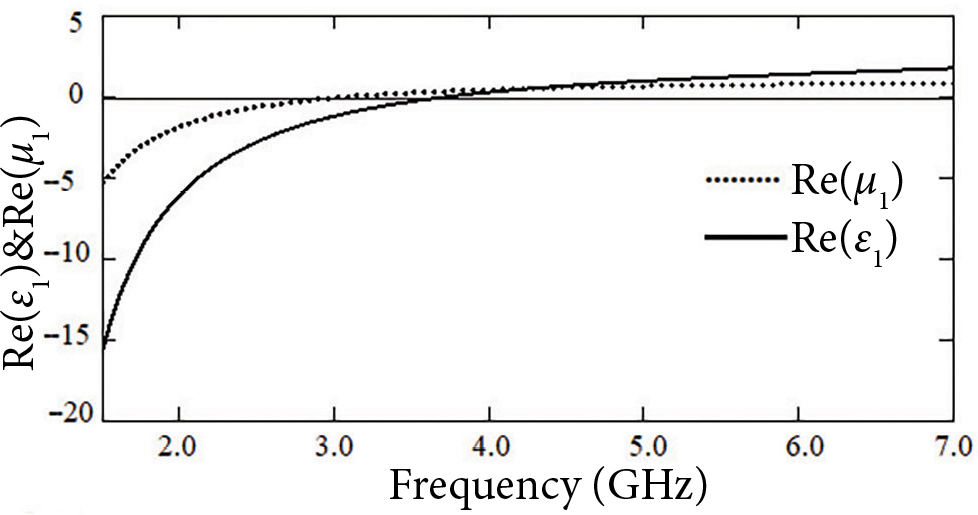Fig. 2. Variation in the real part of permittivity and permeability of DNG metamaterial with frequency at electric and damping frequencies γe = γm = 1 × 10–3 GHz.

First, we investigate the  effect of change in the thickness of DNG metamaterial (dA) and NMP layer (dB), respectively, on the  optical reflectance spectra of APC at the normal incidence for the TE-polarized wave keeping other parameters fixed. Figure 3 shows the  optical reflectance spectra at a different thickness of the DNG material such as dA = 2, 4, 6 and 8 mm, respectively, for the azimuthal mode number m = 2, starting radius ρ0 = 30 mm, electron density of plasma layer ne = 6 × 1017 m–3 and dB = 3 mm. At the right side of the figure, an indicator of colours is shown which represents the  colour dependent reflectance of electromagnetic wave. If colours are close to blue (dark), they show that the  amount of reflectance decreases (transmittance increases), and if they become yellow (light), the  amount of reflectance increases (transmittance decreases). The position of the band gap (BG) centre lies at 3.45 GHz at m = 0 that is equivalent to the BG centre of 1D planar PC and is shown by a dashed line in all the figures. This is so because the geometric difference due to the curved interfaces in APC has no effect on the reflectance properties compared to the 1D planar PC at m = 0.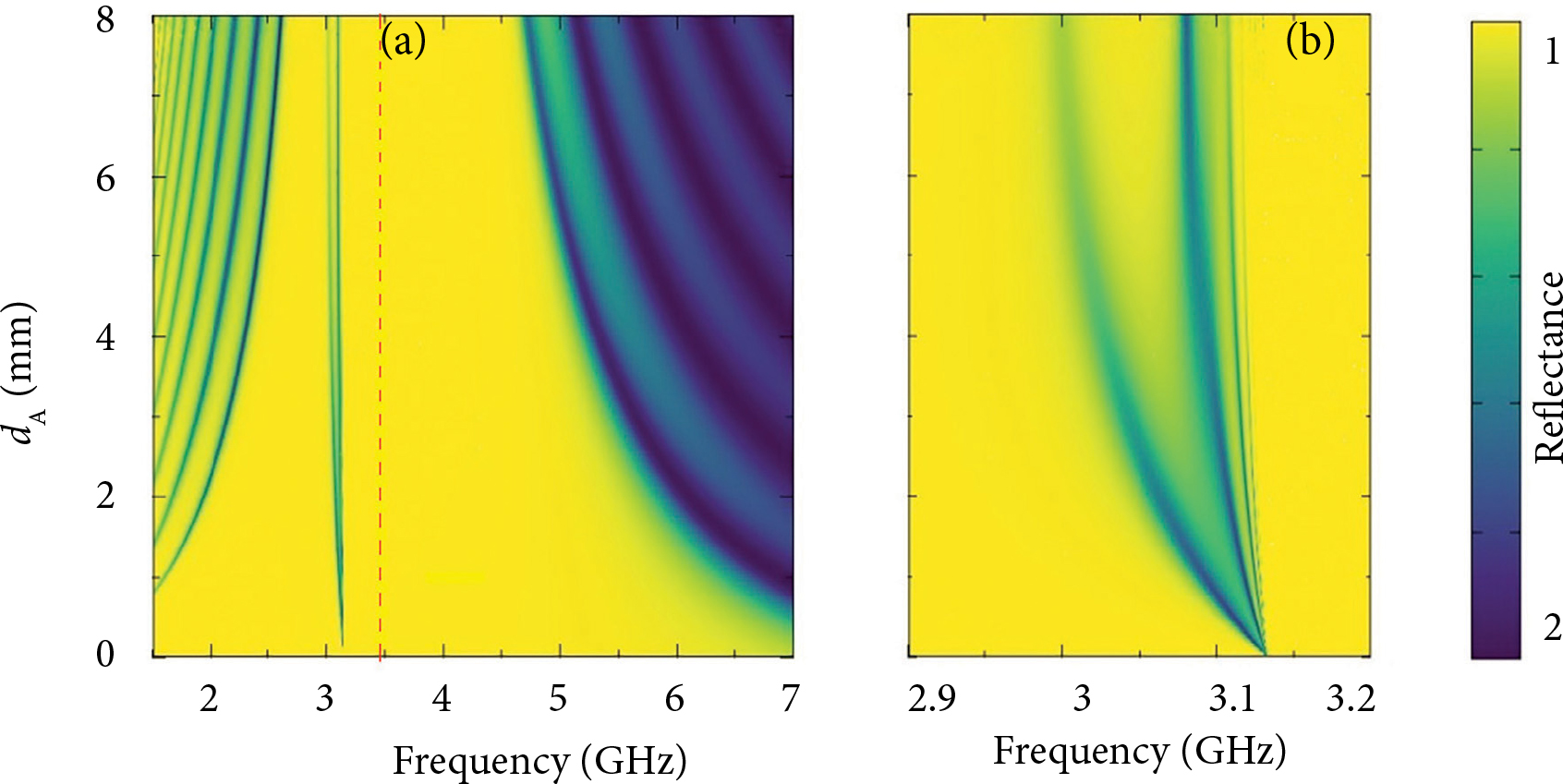Fig. 3. (a) Optical reflectance spectra at dA = 2, 4, 6 and 8 mm, respectively, for azimuthal mode number m = 2, starting radius ρ0 = 30 mm, electron density of plasma layer ne = 6 × 1017 m–3 and dB = 3 mm. (b) Zoom portion of the reflectance panel of (a) in the frequency range 2.9 to 3.2 GHz.

From Fig. 3(a) it can be observed that there exist two reflection bands (photonic band gaps) in a given frequency range for the selected parameters of APC. When the thickness of NMP layer is kept fixed at 3 mm, then by increasing the thickness of DNG metamaterial, the  width of both reflection bands decreases. The left edges of the first PBGs are shifted towards the low frequency side but the right edges remain almost at the same frequency, while in the second PBGs the left band edges lie almost at the same frequency but the right edges shift towards a  higher frequency side. One can say that the first PBG is red-shifted whereas the second PBG is blue-shifted by increasing the thickness of DNG material. The zoom portion of the reflectance panel in the frequency range 2.9 to 3.2 GHz is shown in Fig. 3(b). As can be seen from the panel (3b), there few transmission peaks all have origin at frequency nearly equal to 3.13 GHz where the permeability of metamaterial layer becomes zero. The transmission peaks are very sharp for a  small thickness of the  DNG metamaterial layer but they increase slightly with an increase in the thickness.

Figure 4 demonstrates the  optical reflectance as a  function of frequency at different thicknesses of the NMP layer dB = 2, 4, 6 and 8 mm while the thickness of DNG material is kept fixed at 4 mm. The other parameters are the same as taken above. From the  study of reflectance spectra it is found that the  width of PBGs increases with increasing the  thickness of NMP layer which is opposite to the case when the thickness of DNG material is increased. Further, it is noticed that for smaller values of the thickness (dB < 5 mm) there are two PBGs, one with a  smaller and other with a  larger band width. But when the  thickness of NMP layer becomes greater than 5 mm, both PBGs merge with each other and a very broad reflection band is observed.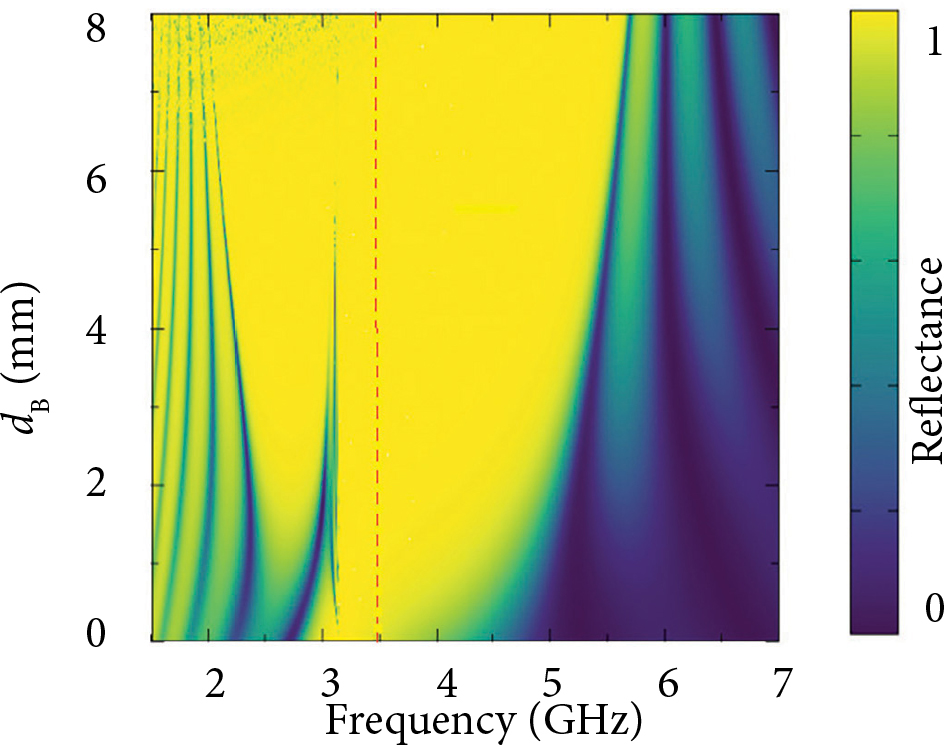Fig. 4. Optical reflectance at dB = 2, 4, 6 and 8 mm, respectively, for azimuthal mode number m = 2, starting radius ρ0 = 30 mm, electron density of plasma layer ne = 6 × 1017m–3 and dA = 4 mm.

The important feature of the  above discussion is that the thickness of both layers has an appreciable influence on the position and width of reflection bands. The  trend of change in PBG with an increase in the thicknesses of the DNG metamaterial and NMP layer is of a different nature and in contrast to each other. Hence, it can be concluded that the presence of NMP layer causes the increase in PBG whereas the DNG layer reduces the PBG when the  thickness of a  respective layer is increased. These results suggest that the annular PC structure consisting of the DNG metamaterial and NMP layer can be used to design a narrow band and a broad band optical reflector in a low and high frequency region by the proper choice of structure parameters.

Now we will investigate the effect of change in the azimuthal mode number m on the reflectance spectra. The azimuthal mode number is an additional parameter for the  APC structure, in comparison to the plane photonic crystal, which controls the  position and width of reflection bands. Since the field solutions of cylindrical Bragg waves depend on the azimuthal mode number for both polarizations, therefore, the reflectance spectra of APC are greatly influenced by changes in the azimuthal mode number.

The optical reflectance for different azimuthal mode numbers m = 0, 1, 2, 3, 4 and 5, respectively, is illustrated in Fig. 5, for the parameters ρ0 = 30 mm, ne = 6 × 1017 m–3, dA = 4 mm and d= 3 mm, respectively. It is obvious from Fig. 5 that for m = 0, there exists a single reflection band but for m > 0 the reflection band gets splitted into two bands separated by a narrow line at the frequency nearly equal to 3.13 GHz. For the TE-reflectance, splitting of PBG occurs at the frequency equal to 3.13 GHz corresponding to the zero permeability value for m > 0. The occurrence of zero-µ gap is due to the radial component of the magnetic field of TE-polarized wave. The first PBG (lies at the lower frequency side) has a much smaller band width than the second PBG which lies at the higher frequency side. The first band lies at nearly 2.3 GHz and the second lies at nearly 4.6 GHz, for m = 0 which is equivalent to the 1D PC case. Further, by increasing the value of m the width of the second PBG increases and is shifted towards the higher frequency side, but in the first PBG band the width remains almost constant for the lower order mode. But for the higher azimuthal mode number, small transmission peaks in the  low frequency range disappear and cause the  broadening in the  PBG. Thus, the  width of the first PBG also increases for the higher azimuthal mode number but it is red-shifted in contrast to the second PBG which is blue-shifted.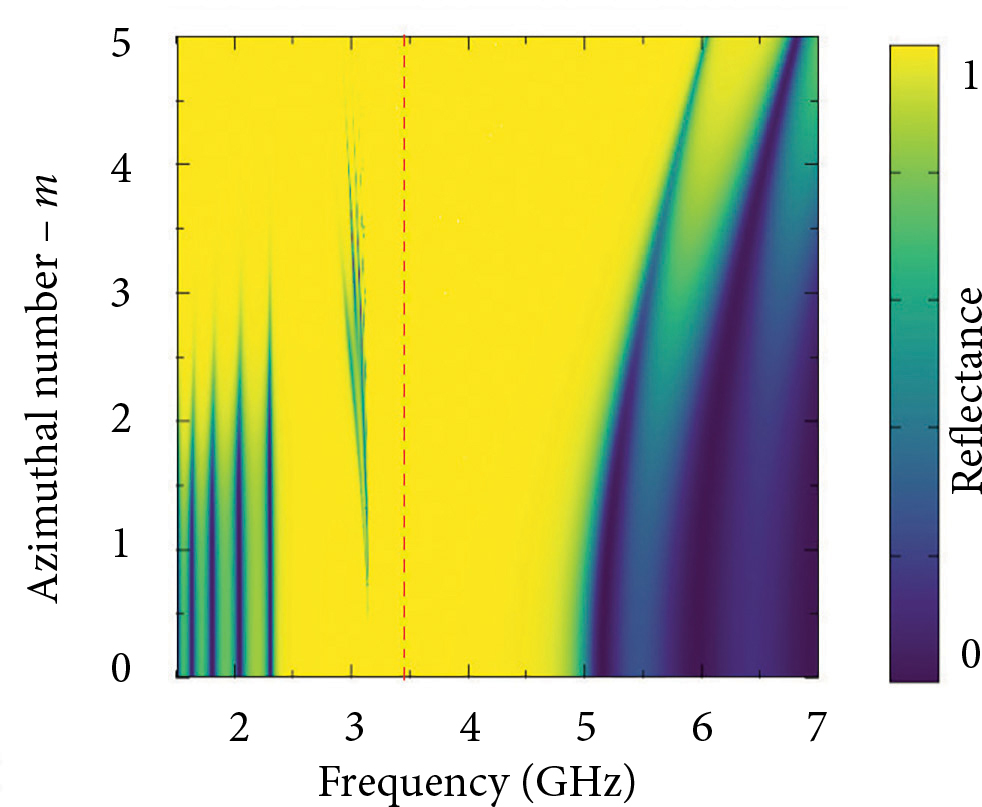Fig. 5. Optical reflectance spectra at different azimuthal mode number m = 0, 1, 2, 3, 4 and 5, for other parameters ρ0 = 30 mm, ne = 6 × 1017m–3, dA = 4 mm and dB = 3 mm, respectively.

One more parameter which controls and affects the optical reflectance properties of APC structure is the  starting radius parameter ρ0 of the  curved surface. The optical reflectance spectra for the parameters m = 2, ne = 6 × 1017m–3, dA = 4 mm and dB  =  3  mm, respectively, at different starting radius ρ0 = 10, 20, 30, 40 and 50 mm, respectively, are illustrated in Fig. 6. On examining the reflectance spectra it can be seen that for a small value of ρ0 = 10 mm, a wider reflection band is observed. But as the value of starting radius increases, the band gets splitted in two parts, similarly to the previous case, by a  narrow boundary lies at the  frequency nearly equal to 1.13 GHz. It is further noticed that the first PBG (lies on the low frequency side) remains almost unaffected by increasing the value of starting radius, but the width of the second PBG (lies on the high frequency side) decreases by increasing the starting radius. Thus, by changing the size of the inner core region, the width of reflection band and its spectral position can be controlled without changing the  other parameters of the  structure. Since the starting radius parameter shows a strong dependence in controlling PBG, therefore it can be considered as a significant parameter (in addition to the thickness of constituent layers) in designing and producing the optical devices.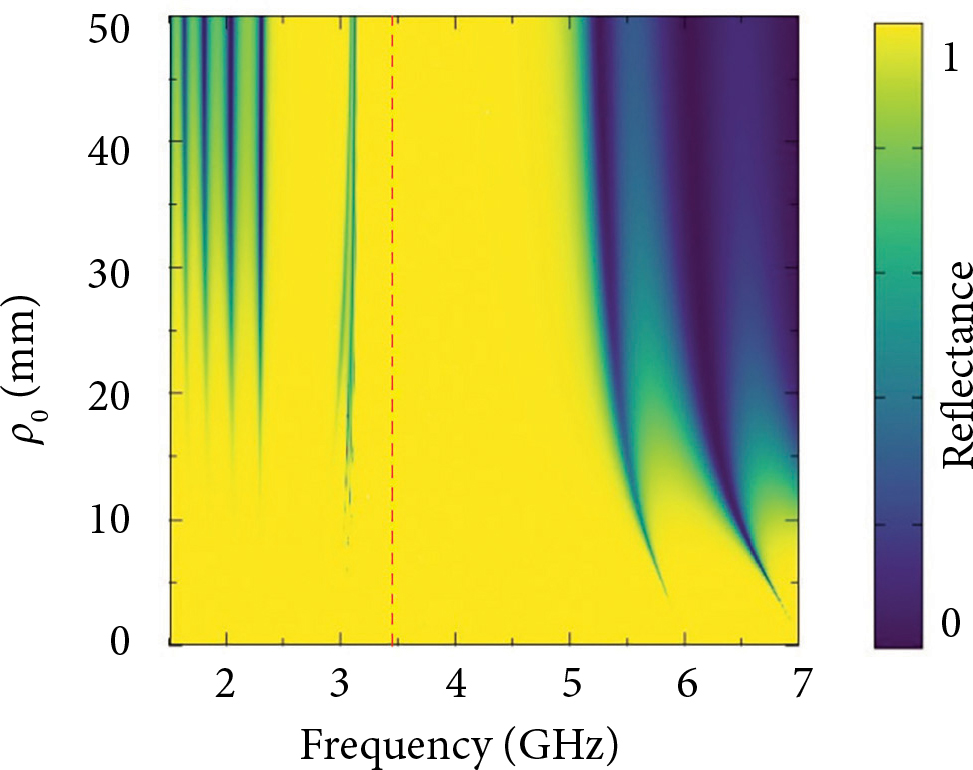Fig. 6. Optical reflectance spectra at different starting radius ρ0 = 10, 20, 30, 40 and 50 mm, respectively, and other parameters m = 2, ne = 6 × 1017m–3, dA = 4 mm and dB = 3 mm, respectively.

Finally, we focus our discussion on the  case of variation in plasma electron density for ne = 2 × 1017, 4 × 1017, 6 × 1017 and 8 × 1017 m–3, respectively, on the  reflectance spectra of APC structure, for the parameters m = 2, ρ0 = 30 mm, d= 4 mm and dB = 3 mm, respectively, as shown in Fig.7. It can be observed from the reflectance spectra that the  width of the  first PBG changes very slightly but the second PBG is greatly influenced by the increase in the plasma electron density. When the electron density of NMP layer increases, the width of the second PBG also increases and it is blue-shifted. The left edge of the second PBG lies at the frequency nearly equal to 3.13 GHz for any value electron density, but the  right edge of the PBG shifts towards the high frequency side. The  enhancement in the  reflection band occurs due to the resultant dielectric constant difference between the DNG metamaterial and NMP layer as the plasma electron density increases. The results predict that the use of NMP layer adds one more parameter for controlling the  width and spectral position of reflection bands of the proposed APC structure.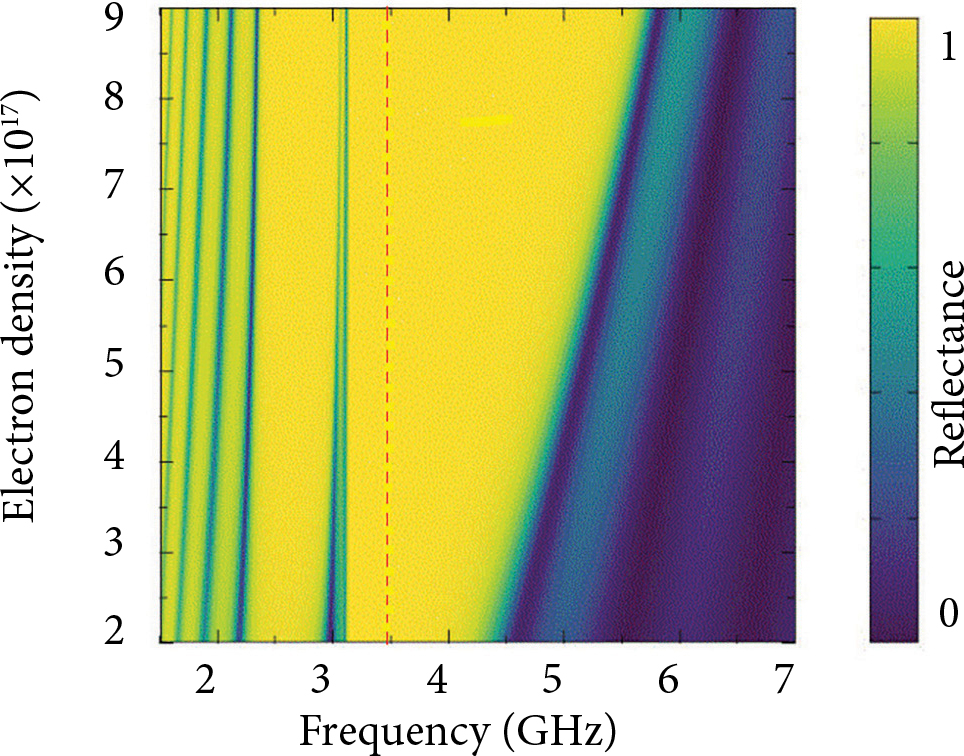Fig. 7. Optical reflectance spectra at different plasma density ne = 2 × 1017, 4 × 1017, 6 × 1017 and 8 × 1017 m–3, respectively, for parameters m  =  2, ρ0  =  30  mm, dA = 4 mm and dB = 3 mm, respectively.

## 4. Conclusions

In summary, we have examined theoretically and studied the  optical reflectance properties of an annular photonic crystal (APC) composed of the alternate layers of double negative (DNG) material and non-magnetized plasma (NMP) layer, immersed in free space. The numerical investigations are demonstrated using the transfer matrix method for cylindrical waves in the  case of TE-polarized wave only. On examining the effect of variation in the  thickness of DNG metamaterial and NMP layer alternately, by keeping other parameters fixed, on the optical reflectance of APC it is found that the  spectral position and width of the  reflection bands are greatly influenced. It is interesting to note that the trends of change in PBG with increase in the thicknesses of the DNG metamaterial and NMP layer are of different nature and opposite to each other. The investigation of increase in the azimuthal mode number m on the  reflectance spectra shows the  appearance of splitting in the PBG when m > 0 and bands occur at the  frequency corresponding to the  zero permeability value of DNG metamaterial layer. Moreover, with an increase in the azimuthal mode number one PBG is red-shifted and the  second one is blue-shifted. In addition to the  above investigation, we also analyzed the effect of increasing the  size of the  starting radius parameter on the  reflectance spectra. The  result predicts that the width of the reflection band and its spectral position can also be controlled by varying the size of the inner core region without changing the other parameters of the structure. Finally, the effect of plasma electron density on the reflectance spectra has been studied. The results show that the reflection band lies in the low frequency region and is affected very little while there is a significant enhancement in the second PBG with an increase in plasma electron density.

From the above discussions it may be inferred that the annular PC structure consisting of DNG metamaterial and NMP layer can be used to design a narrow band and a broad band optical reflector in a low and high frequency region by the proper choice of structure parameters. Moreover, the starting radius can be considered as a significant parameter in designing and producing the optical devices.

## Acknowledgements

One of the  authors, Dr. Sanjeev K Srivastava, is thankful to the Amity Institute of Applied Sciences, Amity University Uttar Pradesh, Noida, India, for providing the necessary facility for this work.

## References

 E. Yablonovitch, Inhibited spontaneous emission in solid-state physics and electronics, Phys. Rev. Lett. 58, 2059 (1987).

 S. John, Strong localization of photons in certain disordered dielectric superlattices, Phys. Rev. Lett. 58, 2486 (1987).

 S.K.  Srivastava and S.P. Ojha, Photonic band gaps in one-dimensional metallic star waveguide structure, Prog. Electromagn. Res. 84, 349 (2008).

 C.J. Wu, Y.H. Chung, B.J. Syu, and T.J. Yang, Band gap extension in a one-dimensional ternary metal-dielectric photonic crystal, Prog. Electromagn. Res. 102, 81 (2010).

. S.M. Weiss, M. Haurylau, and P.M. Fauchet, Tunable photonic bandgap structures for optical interconnects, Opt. Mater. 27, 740 (2005).

 S.K. Srivastava, M. Upadhyay, S.K. Awasthi, and S.P.  Ojha, Tunable reflection bands and defect modes in one-dimensional tilted photonic crystal structure, Opt. Phot. J. 2, 230 (2012).

 H. Ren, C. Jiang, W. Hu, M. Gao, and J. Wang, Photonic crystal channel drop filter with a wavelength-selective reflection micro-cavity, Opt. Express 14, 2446 (2006).

 J.  Zimmermann, M.  Kamp, A.  Forchel, and R. März, Photonic crystal waveguide directional couplers as wavelength selective optical filters, Opt. Commun. 230, 387 (2004).

 O.A. Abd El-Aziz, H.A. Elsayed, and M.I. Sayed, One-dimensional defective photonic crystals for the sensing and detection of protein, Appl. Opt. 58(30), 8309 (2019).

 Y.  Fink, J.N.  Winn, S.  Fan, C.  Chen, J.  Michel, J.D.  Joannopoulos, and E.L.  Thomas, A dielectric omnidirectional reflector, Science 282, 1679 (1998).

 S.K. Srivastava and S.P. Ojha, Operating characteristics of an optical filter in a metallic photonic bandgap materials, Microw. Opt. Technol. Lett. 35, 68 (2002).

 N.R. Ramanujam, I.S. Amiri, S.A. Taya, S. Olyaee, R.  Udaiykumar, A.P.  Pandian, K.S.J.  Wilson, P.  Mahalakshmi, and P.P.  Yupapin, Enhanced sensitivity of cancer cell using one dimensional nano composite material coated photonic crystal, Microsyst. Technol. 25, 189 (2019).

 A.T. Exner, I. Pavlichenko, D. Baierl, M. Schmidt, G. Derondeau, B.V. Lotsch, P. Lugli, and G. Scarpa, A step towards the  electronic nose: integrating 1D photonic crystals with organic light-emitting diodes and photodetectors, Laser Photonics Rev. 8, 726–733 (2014).

 F. Bayat, S.A. Kandjani, and H. Tajalli, Designing real-time biosensors and chemical sensors based on defective 1-D photonic crystals, IEEE Photonics Technol. Lett. 28, 1843 (2016).

 V.G. Veselago, The electrodynamics of substances with simultaneously negative values of ɛ and µ, Sov. Phys. Usp. 10, 509 (1968).

 D.R. Smith, W.J. Padilla, D.C. Vier, S.C. Nemat-Nasser, and S. Schultz, Composite medium with simultaneously negative permeability and permittivity, Phys. Rev. Lett. 84, 4184 (2000).

 J.B. Pendry, Negative refraction makes light run backward in time, Phys. World 13, 27 (2000).

 R.A. Shelby, D.R. Smith, and S. Schultz, Experimental verification of a negative index of refraction, Science 292, 77 (2001).

 J.B. Pendry, Negative refraction makes a perfect lens, Phys. Rev. Lett. 85, 3966 (2000).

 A.  Mishra, S.K.  Awasthi, S.K.  Srivastava, U. Malaviya, and S.P. Ojha, Tunable and omnidirectional filters based on one-dimensional photonic crystals composed of a single-negative materials, J. Opt. Soc. Am. B 28, 1416 (2011).

 S.K.  Srivastava and A.  Aghazamali, Analysis of reflectance properties in 1D photonic crystal containing metamaterial and high-temperature superconductor, J. Supercond. Nov. Magn. 30, 343 (2017).

 J. Li, L. Zhou, C.T. Chan, and P. Sheng, Photonic band gap from a stack of positive and negative index materials, Phys. Rev. Lett. 90, 083901 (2003).

 I.V. Shadrivov, A.A. Sukhorukov, and Y.S. Kivshar, Beam shaping by a periodic structure with negative refraction, Appl. Phys. Lett. 82, 3820 (2003).

 S.A. Ramakrishna, Physics of negative refractive index materials, Rep. Prog. Phys. 68, 449 (2005).

 P.V.  Parimi, W.T.  Lu, P.  Vodo, and S.  Sridhar, Imaging by flat lens using negative refraction, Nature 426, 404 (2003).

 H.  Jiang, H.  Chen, H.  Li, Y.  Zhang, J.  Zi, and S.-Y.  Zhu, Properties of one-dimensional photonic crystals containing single-negative materials, Phys. Rev. E 69, 066607 (2004).

 S.K Srivastava and A. Aghajamali, Narrow transmission mode in one-dimensional symmetric defective photonic crystal containing metamaterial and high Tc superconductor, Opt. Appl. 49, 37 (2019).

 H.-F.  Zhang, S.-B.  Liu, X.-K.  Kong, B.-R.  Bian, and Y. Dai, Omnidirectional photonic band gap enlarged by one-dimensional ternary unmagnetized plasma photonic crystals based on a  new Fibonacci quasiperiodic structure, Phys. Plasmas 19, 112102 (2012).

 C.  Nayak, A.  Aghajamali, T.  Alamfard, and A. Saha, Tunable photonic band gaps in an extrinsic Octonacci magnetized cold plasma quasicrystal, Phys. B Phys. Condens. Matter 525, 41 (2017).

 H.-F. Zhang, S.-B. Liu, X.-K. Kong, C. Chen, and B.-R. Bian, The characteristics of photonic band gaps for three-dimensional unmagnetized dielectric plasma photonic crystals with simple-cubic lattice, Optics Commun. 288, 82 (2013).

 H.F. Zhang, S.-B. Liu, and B.-X. Li, Investigation on the  properties of omnidirectional photonic band gaps in two-dimensional plasma photonic crystals, Phys. Plasmas 23, 12105 (2016).

 M. Heiblum and J.H. Harris, Analysis of curved optical waveguides by conformal transformation, IEEE J. Quantum Electron. 11, 75 (1975).

 E.-X.  Ping, Transmission of electromagnetic waves in planar, cylindrical, and spherical dielectric layer systems and their applications, J. Appl. Phys. 76, 7188 (1994).

 T.  Erdogan and D.G.  Hall, Circularly symmetric distributed feedback laser: coupled mode treatment of TE vector fields, IEEE J. Quantum Electron. 28, 612 (1992).

 J. Scheuer and Y. Yariv, Coupled-waves approach to the design and analysis of Bragg and photonic crystal annular resonators, IEEE J. Quantum Electron. 39, 1555 (2003).

 M. Toda, Single-mode behavior of a circular grating for potential disk-shaped DFB lasers, IEEE J. Quantum Electron. 26, 473 (1990).

 M.  Fallahi, F.  Chatenoud, I.M.  Templeton, M.  Dion, C.M.  Wu, A.  Delage, and R.  Barber, Electrically pumped circular-grating surfaceemitting DBR laser on InGaAs strained singlequantum-well structure, IEEE Photon. Tech. Lett. 4, 1087 (1992).

 T.  Erdogan, O.  King, G.W.  Wicks, D.G.  Hall, E.H.  Anderson, and M.J.  Rooks, Circularly symmetric operation of a concentric-circle-grating, surface-emitting, AlGaAs/GaAs quantum-well semiconductor laser, Appl. Phys. Lett. 60, 1921 (1992).

 W.M.J. Green, J. Scheuer, G. DeRose, and Y. Yariv, Vertically emitting annular Bragg lasers using polymer epitaxial transfer, Appl. Phys. Lett. 85, 3669 (2004).

 J. Scheuer and Y. Yariv, Two-dimensional optical ring resonators based on radial Bragg resonance, Opt. Lett. 28, 1528 (2003).

 J.  Scheuer and Y.  Yariv, Annular Bragg defect mode resonators, J. Opt. Soc. Am. B 20, 2285 (2003).

 J. Scheuer, W.M.J. Green, G. DeRose, and Y. Yariv, Low-threshold two-dimensional annular Bragg lasers, Opt. Lett. 29, 2641 (2004).

 M.A. Kaliteevski, R.A. Abram, V.V. Nikolaev, and G.S.  Sokolovski, Bragg reflectors for cylindrical waves, J. Mod. Opt. 46, 875 (1999).

 A.  Aghajamali, T.  Alamfard, and C.  Nayak, Investigation of reflectance properties in a symmetric defective annular semiconductor–superconductor photonic crystal with a  radial defect layer, Phys. B Condens. Matter 605, 412770-1 (2021).

 S.K. Srivastava and A. Aghajamali, Investigation of reflectance properties in 1D ternary annular photonic crystal containing semiconductor and high-Tsuperconductor, J. Supercond. Nov. Magn. 29, 1423 (2016).

 M.S. Chen, C.J. Wu, and T.J. Yang, Optical properties of a semiconducting annular periodic multilayer structure, Solid State Commun. 149, 1888 (2009).

 C.A.  Hu, C.J.  Wu, T.J.  Yang, and S.L.  Yang, Analysis of optical properties in cylindrical dielectric photonic crystal, Opt. Commun. 291, 424 (2013).

 T.W. Chang, H.T. Hsu, and C.J. Wu, Investigation of photonic band gap in a circular photonic crystal, J. Electromag. Waves Appl. 25, 2222 (2011).

 S.K. Srivastava and A. Aghajamali, Study of optical reflectance properties in 1D annular photonic crystal containing double negative (DNG) metamaterials, Phys. B Condens. Matter 489, 67 (2016).

 S. Gandhi, S.K. Awasthi, and A.H. Aly, Biophotonic sensor design using a 1D defective annular photonic crystal for the  detection of creatinine concentration in blood serum, RSC Adv. 11, 26655 (2011).

 L. Shiveshwari and P. Mahto, Photonic band gap effect in one-dimensional plasma dielectric photonic crystals, Solid State Commun. 138, 160 (2006).

### VIENMACIO ŽIEDINIO FOTONINIO KRISTALO IŠ NEIGIAMU SKVARBU MEDŽIAGOS IR NEIMAGNETINTOS PLAZMOS ATSPINDŽIO JUOSTU TYRIMAS

A. Aghajamali a, S. K. Srivastava b, C. Nayak cd

a Kertino universiteto Fizikos ir astronomijos departamentas, Pertas, Australija

b Utar Pradešo Amity universiteto Taikomuju mokslu institutas, Noida, Indija

c SRM Mokslo ir technologijos instituto Elektronikos ir komunikaciju inžinerijos departamentas, Cenajus, Indija

d Veloro technologijos instituto Elektronikos inžinerijos mokykla, Veloras, Indija